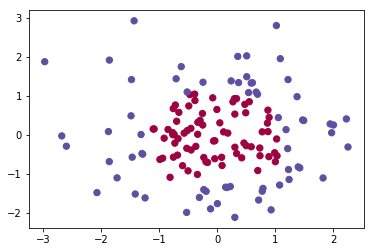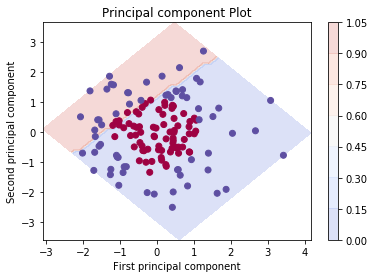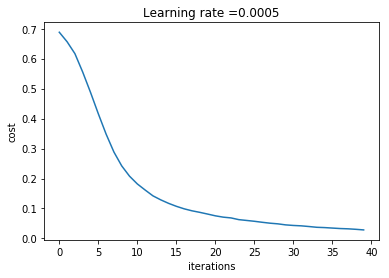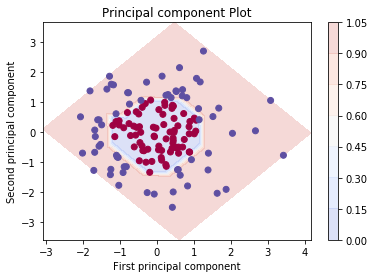# Deep Neural Network with TensorFlow¶

### Elan Ding¶

Modified: July 22, 2018

We will create a deep neural network using the TensorFlow library. If you have read my previous post, and saw how a deep neural network was implemented in numpy, this post will be a lot easier to follow! This is due to the awesome features of TensorFlow that automates a lot of tasks for us. Before we get started, let's import all the libraries we need.

In :
import math
import numpy as np
import pandas as pd
import matplotlib.pyplot as plt
import sklearn.datasets
from sklearn.model_selection import train_test_split
import tensorflow as tf
from tensorflow.python.framework import ops
from elan.ML import encode_one_hot, pca_contour, standardize, decode_one_hot, glm
# Note that elan.ML is my own library


We make a dataset using sklearn.dataset.gaussian_quantiles. This method uses a multivariate Gaussian distribution and separate into classes by intersecting it with concentric hyperspheres.

In :
# Create a dataset
N = 200
gaussian_quantiles = sklearn.datasets.make_gaussian_quantiles(mean=None, cov=0.5, n_samples=N,
n_features=2, n_classes=2,
shuffle=True, random_state=None)
return gaussian_quantiles

In :
# Load the data set
data = gauss
label = gauss

# Train test split
X_train_orig, X_test_orig, Y_train_orig, Y_test_orig = train_test_split(data, label, test_size=0.3, random_state=1)

# Reshape and standardize
X_train = standardize(X_train_orig).T
X_test = standardize(X_test_orig).T

# one hot encoder
Y_train = encode_one_hot(Y_train_orig.T)
Y_test = encode_one_hot(Y_test_orig.T)

print("Training data has dimension {}".format(X_train.shape))
print("Training label has dimension {}".format(Y_train.shape))
print("Testing data has dimension {}".format(X_test.shape))
print("Testing label has dimension {}".format(Y_test.shape))

Training data has dimension (2, 140)
Training label has dimension (2, 140)
Testing data has dimension (2, 60)
Testing label has dimension (2, 60)

In :
# Visualize the data
plt.scatter(X_train[0, :], X_train[1, :], c=Y_train_orig, s=40, cmap=plt.cm.Spectral)

Out:
<matplotlib.collections.PathCollection at 0x1a2ca4f6a0>Given the circular shape, we know that linear model lacks the flexibility to learn it. Let's try logistic regression.

In :
logmodel, _ = glm(X_train.T, Y_train_orig)

The 5-fold cross validation score is (0.389812987815839, 0.5959682515091593)

In :
# Plot decision boundary
pca_contour(logmodel.predict, X_train.T, Y_train_orig, 50, zoom=0)

Assuming that model is fit with standardized data.Clearly logistic regression is a poor choice here. Let's do better!

## Neural Network with TensorFlow¶

Let's build a deep neural network using TensorFlow. All variables in TensorFlow must be declared. So we first create the placeholders for data and label as matrices of dimension $(n_x, m)$ and $(n_y, m)$ where $n_x$ is the number of features, $n_y$ is the number of classes, and $m$, specified as None, denotes the number of training examples.

In :
def create_placeholders(n_x, n_y):

X = tf.placeholder("float", [n_x, None])
Y = tf.placeholder("float", [n_y, None])

return X, Y


Our neural network can have arbitrary dimensions. Here we specify it as a 3 layer network whose dimension is given by the list layers_dims.

In :
tf.reset_default_graph()
layers_dims = [2, 25, 12, 2]


Next we initialize the parameters using Xavier initialization for the weights and zero initialization for the bias.

In :
def initialize_parameters(layers_dims):

tf.set_random_seed(2)

parameters = {}
L = len(layers_dims) - 1

for l in range(1, L + 1):

parameters['W' + str(l)] = tf.get_variable("W"+str(l), [layers_dims[l], layers_dims[l-1]], initializer=tf.contrib.layers.xavier_initializer(seed=1))
parameters['b' + str(l)] = tf.get_variable("b"+str(l), [layers_dims[l], 1], initializer=tf.zeros_initializer())

return parameters


As usual, we need to define forward propagation. The input X in forward_propagation must be a matrix of dimension $(n_x, m)$ and parameters is the output of initialize_parameters function defined above.

In :
def forward_propagation(X, parameters):

L = len(parameters) // 2
A = X

for l in range(1, L):
A_prev = A

A = tf.nn.relu(Z)

return ZL


Here is when things become simple. TensorFlow has a built-in function to compute the cost function. In this example we only have two classes. For multi-class classification, use softmax_cross_entropy_with_logits_v2 instead.

In :
def compute_cost(ZL, Y):

logits = tf.transpose(ZL)
labels = tf.transpose(Y)

cost = tf.reduce_mean(tf.nn.sigmoid_cross_entropy_with_logits(logits=logits, labels=labels))

return cost


For large datasets, gradient descent can be very slow. Here we define a function that first shuffles the data set, and divides it into mini-batches. Instead of running on the entire data set, the gradient optimizer runs on each of the mini-batches and update the parameters accordingly. This is called mini-batch gradient descent. The function random_mini_batches below returns a list of paired mini batches (mini_batch_X, mini_batch_Y), so we can feed them into our model later.

In :
def random_mini_batches(X, Y, mini_batch_size = 64, seed = 0):

m = X.shape
mini_batches = []
np.random.seed(seed)

# Shuffle the data set
permutation = list(np.random.permutation(m))
shuffled_X = X[:, permutation]
shuffled_Y = Y[:, permutation].reshape((Y.shape,m))

# Partition the data set, except for the last partition
num_complete_minibatches = math.floor(m/mini_batch_size)
for k in range(0, num_complete_minibatches):
mini_batch_X = shuffled_X[:, k * mini_batch_size : k * mini_batch_size + mini_batch_size]
mini_batch_Y = shuffled_Y[:, k * mini_batch_size : k * mini_batch_size + mini_batch_size]
mini_batch = (mini_batch_X, mini_batch_Y)
mini_batches.append(mini_batch)

# The last batch
if m % mini_batch_size != 0:
mini_batch_X = shuffled_X[:, num_complete_minibatches * mini_batch_size : m]
mini_batch_Y = shuffled_Y[:, num_complete_minibatches * mini_batch_size : m]
mini_batch = (mini_batch_X, mini_batch_Y)
mini_batches.append(mini_batch)

return mini_batches


Great news: We don't need to implement back propagation. TensorFlow takes care of it automatically. So we are ready to build our model.

In :
def model(X_train, Y_train, X_test, Y_test, layers_dims, learning_rate = 0.0005,
num_epochs = 700, minibatch_size = 32, print_cost = True):

ops.reset_default_graph()
tf.set_random_seed(1)
seed = 66
(n_x, m) = X_train.shape
n_y = Y_train.shape
costs = []

X, Y = create_placeholders(n_x, n_y)

parameters = initialize_parameters(layers_dims)

ZL = forward_propagation(X, parameters)

cost = compute_cost(ZL, Y)

init = tf.global_variables_initializer()

with tf.Session() as sess:

sess.run(init)

for epoch in range(num_epochs):

epoch_cost = 0.
num_minibatches = int(m / minibatch_size)
seed = seed + 1
minibatches = random_mini_batches(X_train, Y_train, minibatch_size, seed)

for minibatch in minibatches:

(minibatch_X, minibatch_Y) = minibatch

_ , minibatch_cost = sess.run([optimizer, cost],
feed_dict={X: minibatch_X,
Y: minibatch_Y})

epoch_cost += minibatch_cost / num_minibatches

if print_cost == True and epoch % 100 == 0:
print ("Cost after epoch %i: %f" % (epoch, epoch_cost))
if print_cost == True and epoch % 5 == 0:
costs.append(epoch_cost)

plt.plot(np.squeeze(costs))
plt.ylabel('cost')
plt.xlabel('iterations')
plt.title("Learning rate =" + str(learning_rate))
plt.show()

parameters = sess.run(parameters)

correct_prediction = tf.equal(tf.argmax(ZL), tf.argmax(Y))

accuracy = tf.reduce_mean(tf.cast(correct_prediction, "float"))

print ("Train Accuracy:", accuracy.eval({X: X_train, Y: Y_train}))
print ("Test Accuracy:", accuracy.eval({X: X_test, Y: Y_test}))

return parameters

In :
tf.reset_default_graph()
parameters = model(X_train, Y_train, X_test, Y_test, layers_dims = layers_dims, num_epochs = 200, minibatch_size = 4)

Cost after epoch 0: 0.688056
Cost after epoch 100: 0.075496Train Accuracy: 1.0
Test Accuracy: 0.93333334


That literally took only 2 seconds to train! Deep learning is definitely an overkill for this problem. Next we define a function that make predictions based on the trained parameters.

In :
def predict(X, parameters):

L = len(parameters) // 2
params = {}

for l in range(L):
params['W'+str(l+1)] = tf.convert_to_tensor(parameters['W'+str(l+1)])
params['b'+str(l+1)] = tf.convert_to_tensor(parameters['b'+str(l+1)])

x = tf.placeholder("float", [X.shape, X.shape])

ZL = forward_propagation(x, params)
p = tf.argmax(ZL)

sess = tf.Session()
prediction = sess.run(p, feed_dict = {x: X})
sess.close()

return prediction


To get a visualization of our model, we define a new function that transforms one-hot encoded outputs back to a single vector of outputs.

In :
def decode_one_hot(Y_mat):
'''
Decode a matrix of dimension (C, m)
where C is the number of classes
to a vector Y of shape (1, m)
'''
Y = np.argmax(Y_mat, axis = 0)
return Y

In :
Y_train_numeric = decode_one_hot(Y_train)

In :
pca_contour(lambda x: predict(x.T, parameters), X_train.T, Y_train_numeric.T, pixel_density = 20, zoom=0)

Assuming that model is fit with standardized data.And the graph looks beautiful. :)